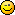Author Topic: iPad orientation / iPhone  (Read 3867 times)Kitty Hello

• code monkey
• Prof. Inline
••• Posts: 10816
• here on my island the sea says 'hello'« on: 2010-Jun-25 »
Code: (glbasic) [Select]
// my_drawangle - angle you can pass to ROTOSPRITE for correct orientation
//
// return:
// 0 = bottom is at bottom
// 1 = button is left
// 2 = button is on top
// 3 = button is right
LOCAL fx, fy
STATIC last%, drawangle
fx = GETJOYX(0)
fy = GETJOYY(0)

LOCAL limit = .75
IF fy < -limit THEN last = 0
IF fx >  limit THEN last = 1
IF fy >  limit THEN last = 2
IF fx < -limit THEN last = 3

LOCAL angle = last * 90

IF ABS(angle - drawangle)>180.0
IF angle < drawangle
INC angle, 360.0
ELSE
DEC angle, 360.0
ENDIF
ENDIF

my_drawangle = drawangle * 0.9 + angle*0.1; drawangle=my_drawangle

RETURN last
ENDFUNCTION
« Last Edit: 2010-Jun-25 by Kitty Hello »Dark Schneider« Reply #1 on: 2011-Aug-08 »
That is not exactly correct, the return values are wrong (the hurry).
Also it can be slighty modified to allow user to select the limit, the final function could be like:

Code: (glbasic) [Select]
// --------------------------------- //
// my_drawangle - angle you can pass to ROTOSPRITE for correct orientation
//
// return:
// 0 = bottom is at right
// 1 = button is bottom
// 2 = button is on left
// 3 = button is top
LOCAL fx, fy
STATIC last%, drawangle
fx = GETJOYX(0)
fy = GETJOYY(0)

IF fy < -limit THEN last = 0
IF fx >  limit THEN last = 1
IF fy >  limit THEN last = 2
IF fx < -limit THEN last = 3

LOCAL angle = last * 90

IF ABS(angle - drawangle)>180.0
IF angle < drawangle
INC angle, 360.0
ELSE
DEC angle, 360.0
ENDIF
ENDIF

my_drawangle = drawangle * 0.9 + angle*0.1; drawangle=my_drawangle

RETURN last
ENDFUNCTION

Very good function